## Thursday, June 09, 2011

### Series-Parallel Circuits- Part 2

This is just the continuation of my post yesterday about Series-Parallel Circuits- Part 1. I've already provided you the steps on how to simplify a simple series-parallel connections. Today, I will give you an example on how to solve that circuit using that steps mentioned before.

The practical example that I will show you below is how to break down a complex circuits to find the total resistance. Refer to figure below: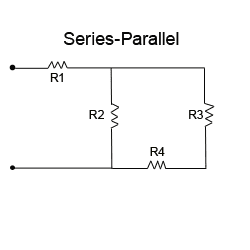Circuit Problem for Series-Parallel
Let's say:  R1= 7 ohms, R2= 10 ohms, R3= 6 ohms and R4= 4 ohms. We are required to get the total resistance of the circuit.

Using the steps that previously discussed here. We can redraw an equivalent circuit in a way that we can understand it well. The figure below is the redrawn circuit for the given problem above.

1. Redraw the circuit.
﻿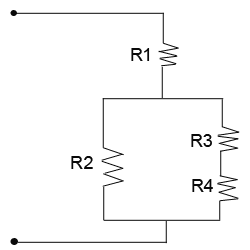Redrawn Series-Parallel
From the redrawn circuit above. we can now simplify R3 and R4. Lets name it R3-4 = 6+4 = 10 ohms.

2. The next step is by getting the resistance between R2 and R3-4 connected in parallel. The circuit now will be simplify as shown below:
﻿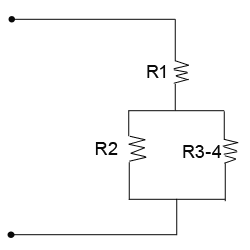Simplified R3-4 to be combine with R2
Next, we will simplify the R2 and R3-4 using the formula of two resistances connected in parallel. Let's name it Ra= R2 X R3-4 / R2 + R3-4 = 10 x 10 / 10 +10 = 5 ohms.

3. Now, take a look on the next circuit figure below.  The circuit was already simplified into series circuit and we can already get the total resistance of the circuit.
﻿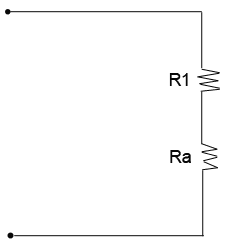Equivalent Simplified Circuit
The total resistance of the given circuit based on the simplified circuit above would be: Rt= R1 + Ra = 7 + 5 = 12 ohms. Very easy right?

It only means that a complex circuit can be broken down into simplified circuit to get the total resistance Rt = 12 ohms.

I will leave the next circuit as your exercise. This is a bit complicated than above circuit problem. The application is still the same. Given that R1= 1 ohm, R2= 2 ohms, R3= 3 ohms, R4= 4 ohms, R5= 5 ohms, R6= 6 ohms, R7= 7 ohms, R8= 8 ohms and R9= 9 ohms. What will be the total resistance of the circuit below?
﻿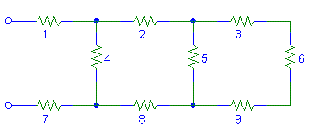Exercise: What is the total resistance of the given circuit?
After further simplication, I found out that the total resistance of the given circuit above is 11.10 ohms. Did you get the same answer? If not, you may leave your comment below and let's discuss...

Since you've already understand the concept of series-parallel circuits, it is now time to move fast on another topic on my next post. This is already the Part 3 and would be dealing with bridge resistor circuits.

Stay tune!

Cheers!